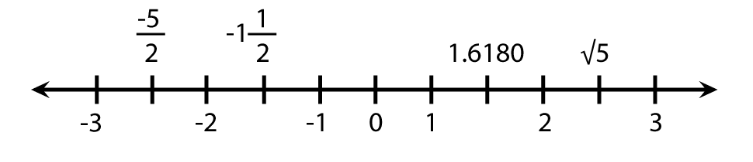# Real Numbers

While preparing for the Quantitative reasoning section in GRE, Real numbers contribute to a significant part of the GRE syllabus. This article would help in understanding some of the concepts of Real numbers.

Want to understand the concepts of real numbers?

Let’s play a game. Imagine any number, any number that you can think of; now ask whether it is a real number or not. The answer you will be getting is YES. Amazed? This is the truth; real numbers have an infinite range that can include any number within it. Imagine real numbers as a superset, whose subsets are natural numbers, whole numbers, rational and irrational numbers, integers, fractions and decimals. The only number that is not a part of the real number is the imaginary number; which is√−1.

Real numbers can be represented on the real number line.$\frac{1}{2} > \frac{-1}{2}$

$\frac{\sqrt{3}}{2} > \frac{1}{2}$

$-1 < 0 < \sqrt{982}$

Every real number corresponds to a point on the number line, and every point on the number line corresponds to a real number. On the number line, all numbers to the left of 0 are negative and all numbers to the right of 0 are positive. Only the number 0 is neither negative nor positive.

## Properties of Real Numbers

• $p + q = q + p$
• $pq = qp$
• $(p + q) + r = p + (q + r)$
• $(pq)r = p(qr)$
• $p (q + r) = pq + qr$
• $p + 0 = p$
• $(p)(0) = 0$
• $(p)(1) = p$
• If pq = 0, then either p=0 or q=0, or both, p, q = 0.
• Dividing any number by 0 is not defined
• Both, p + q and pq will be positive if p and q is positive.
• Both, p + q and pq will be negative if p and q is negative.
• pq will be negative if either p or q is negative
• Triangle Inequality: $|p + q| \leq |p| + |q|$
• $|p||q| = |pq|$
• $if \; p > 1, then \; p^{2} > p$
• $if \; 0 < p < 1, then \; p^{2} < p$

These properties of Real numbers would assist you in your preparation in the GRE Quantitative Reasoning section.

BYJU’S will be glad to help you in your GRE preparation journey. You can ask for any assistance related to GRE from us by just giving a missed call at +918884544444, or you can drop an SMS. You can write to us at gre@byjus.com.